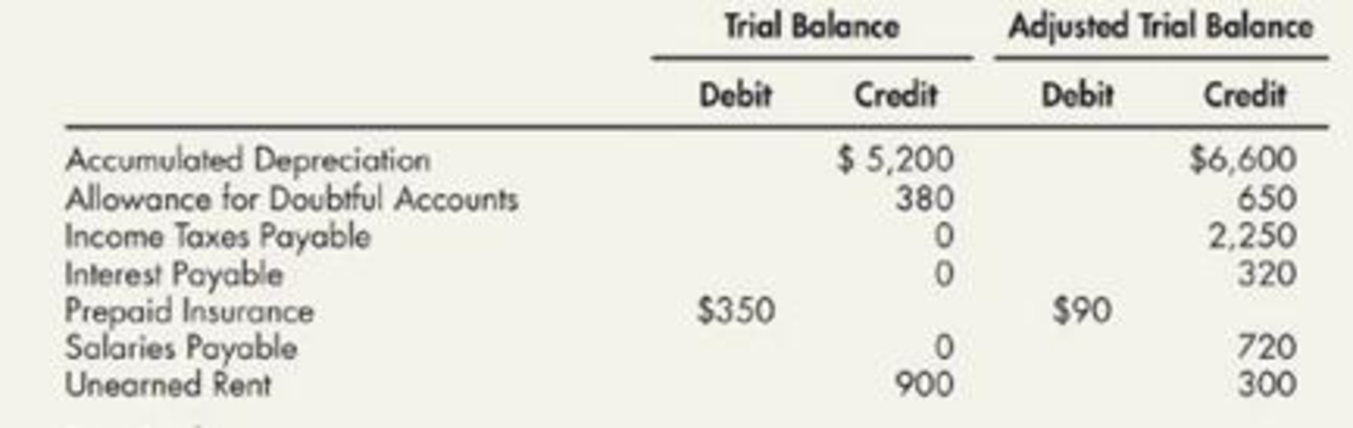Chapter 3, Problem 6E### Intermediate Accounting: Reporting...

3rd Edition
James M. Wahlen + 2 others
ISBN: 9781337788281

#### Solutions

Chapter
Section### Intermediate Accounting: Reporting...

3rd Edition
James M. Wahlen + 2 others
ISBN: 9781337788281
Textbook Problem
34 views

# Adjusting Entries The following partial list of accounts and account balances has been taken from the trial balance and the adjusted trial balance of Baye Company:Required:Next Level Prepare the adjusting entry that caused the change in each account balance.

To determine

Prepare the adjusting entries that would be caused the change in given account balance.

Explanation

Adjusting entries: Adjusting entries are those entries which are recorded at the end of the year, to update the income statement accounts (revenue and expenses) and balance sheet accounts (assets, liabilities, and stockholders’ equity) to maintain the records according to accrual basis principle.

Prepare the adjusting entries that would be caused the change in given account balance as follows:

Accumulated depreciation:

 Date Account Title and Explanation Debit ($) Credit ($) December 31 Depreciation expense 1,400 Accumulated depreciation-Equipment ($6,600−$5,200) 1,400 (To record the depreciation expense incurred at the end of the accounting year)

Table (1)

• Depreciation expense is component of shareholders’ equity, and it decreases the value of shareholders equity. Hence, debit the depreciation expense with $1,400. • Accumulated depreciation is a contra-asset account, and it decreases the value of assets. Hence, credit the accumulated depreciation expense with$1,400.

Allowance for doubtful accounts:

 Date Account Title and Explanation Debit ($) Credit ($) December 31 Bad debt expense 270 Allowance for doubtful accounts ($650−$380) 270 (To record the bad debts expense estimated at the end of the accounting year)

Table (2)

• Bad expense is component of shareholders’ equity, and it decreases the value of shareholders equity. Hence, debit the bad expense with $270. • Allowance for doubtful accounts is a contra-asset account, and it decreases the value of assets. Hence, credit the allowance for doubtful account with$270.

Income tax payable:

 Date Account Title and Explanation Debit ($) Credit ($) December 31 Income tax expense 2,250 Income tax payable ($2,250−$0) 2,250 (To record the income tax expense accrued at the end of the accounting year)

Table (3)

• Income tax expense is component of shareholders’ equity, and it decreases the value of shareholders equity. Hence, debit the interest expense with $2,250. • Income tax payable is a liability account and it increases in the value of liabilities. Hence, credit the income tax payable with$2,250.

Interest payable:

 Date Account Title and Explanation Debit ($) Credit ($) December 31 Interest expense 320 Interest payable ($320−$0) 320 (To record the interest expense accrued at the end of the accounting year)

Table (4)

• Interest expense is component of shareholders’ equity, and it decreases the value of shareholders equity

### Still sussing out bartleby?

Check out a sample textbook solution.

See a sample solution

#### The Solution to Your Study Problems

Bartleby provides explanations to thousands of textbook problems written by our experts, many with advanced degrees!

Get Started

#### In what way is a college degree a form of capital?

Brief Principles of Macroeconomics (MindTap Course List)

#### How should the capital structure weights used to calculate the WACC be determined?

Fundamentals of Financial Management, Concise Edition (MindTap Course List)

#### Once again we can use the CAPM to estimate MMMs cost of equity. From the Internet, you can find a number of dif...

Fundamentals of Financial Management, Concise Edition (with Thomson ONE - Business School Edition, 1 term (6 months) Printed Access Card) (MindTap Course List)